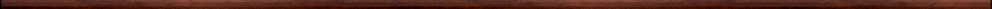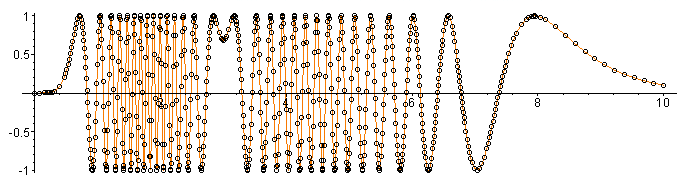Miscellaneous Maple worksheetsIntroductory, general and miscellaneous worksheets:

They are all compatible with Classic Worksheet Maple 10.Prime Numbers - primes.mws

• What are prime numbers?
• Finding prime numbers
• Fermat primes
• Mersenne primes
• The distribution of prime numbers
• Finding large primes
• Twin primes

An adaptive graph plotting procedure - graph.mws

• An adaptive graph plotting procedure: graph.

Solving equations - solve.mws

• Linear equations
• Simultaneous equations in two variables